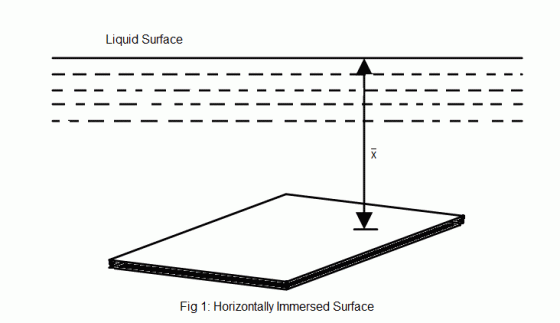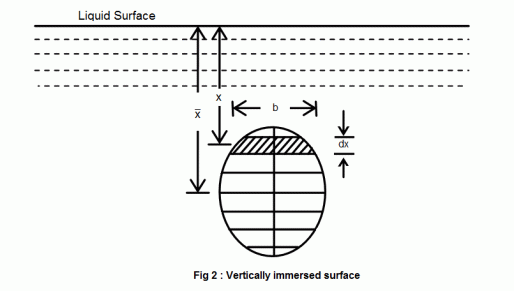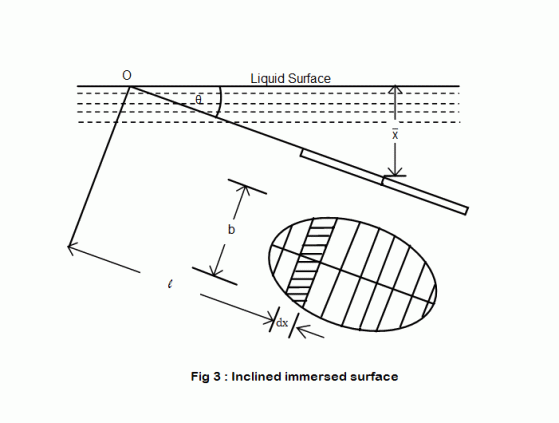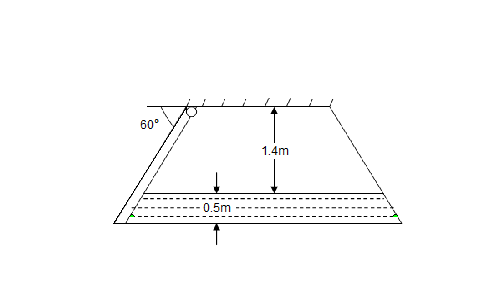•http://facebook.com/
•https://www.google.com/accounts/o8/id
•https://me.yahoo.com

# Total Pressure

Total Pressure on an Immersed Surface

## Overview

The Total pressure on an immersed surface, may be defined as the total pressure exerted by the liquid on it. Mathematically total pressure,
where,
• = Intensities of pressure on different strips of the surface, and
• = Areas of corresponding strips.

The position of an immersed surface may be,
• Horizontal
• Vertical
• Inclined

## Total Pressure On A Horizontal Immersed Surface

Consider a plane horizontal surface immersed in a liquid as shown in figure 1.• = Specific weight of the liquid
• = Area of the immersed surface in
• = Depth of the horizontal surface from the liquid level in meters

We know that the Total pressure on the surface,

P = Weight of the liquid above the immersed surface

= Specific weight of liquid * Volume of liquid

= Specific weight of liquid * Area of surface * Depth of liquid

= kN

Example:
[metric]
##### Example - Total Pressure on a Horizontal Immersed Surface
Problem
A tank 3m 4m contains 1.2m deep oil of specific gravity 0.8. Find

(a) intensity of the pressure at the base of the tank, and

(b) total pressure on the base of the tank.
Workings
Given,
• Area of tank, = 3m 4m = 12m2
• Depth of oil, = 1.2m
• Specific gravity of oil = 0.8

Specific weight of oil, = =

(a) Intensity of pressure at the base of the tank

(b) Total pressure on the base of the tank
Solution
Intensity of pressure at the base of the tank = 9.42 KPa

Total pressure on the base of the tank = 113.4 KN

## Total Pressure On A Vertically Immersed Surface

Consider a plane vertical surface immersed in a liquid shown in figure 2.Let the whole immersed surface is divided into a number of small parallel stripes as shown in figure.

Here,
• = Specific weight of the liquid
• = Total area of the immersed surface
• = Depth of the center of gravity of the immersed surface from the liquid surface

Now, consider a strip of thickness dx, width b and at a depth x from the free surface of the liquid.

The intensity of pressure on the strip =

and the area of strip =

Pressure on the strip = Intensity of pressure * Area =

Now, Total pressure on the surface,

But, = Moment of the surface area about the liquid level =

Example:
[metric]
##### Example - Total Pressure On A Vertically Immersed Surface
Problem
A circular door of 1m diameter closes an opening in the vertical side of a bulkhead, which retains sea water. If the center of the opening is at a depth of 2m from the water level, determine the total pressure on the door. Take specific gravity of sea water as 1.03.
Workings
Given,
• d = 1m
• = 2m
• specific gravity of sea water = 1.03

Specific weight of sea water, = = 10.1 KN/m3

Surface area of the circular door,

Total pressure on the door,

Solution
Total pressure on the door = 15.9 KN

## Total Pressure On An Inclined Immersed Surface

Consider a plane inclined surface, immersed in a liquid as shown in figure 3.Let the whole immersed surface is divided into a number of small parallel stripes as shown in figure.

Here,
• = Specific weight of the liquid
• = Total area of the immersed surface
• = Depth of the center of gravity of the immersed surface from the liquid surface
• = Angle at which the immersed surface is inclined with the liquid surface

Now, consider a strip of thickness dx, width b and at a distance from O (A point on the liquid surface where the immersed surface will meet, if produced).

So, The intensity of pressure on the strip =

and the area of strip =

Pressure on the strip = Intensity of pressure * Area =

Now, Total pressure on the surface,

But, = Moment of the surface area about O =

Example:
[metric]
##### Example - Total Pressure on an Inclined Immersed Surface
Problem
A horizontal passage 1400mm 1400mm has its outlet covered by a plane flap inclined at with the horizontal and its hinged along the upper horizontal edge of the passage. If the depth of the flowing water is 500mm in the passage, determine the thrust on the gate.Workings
Given,
• Width of passage = 1400mm = 1.4m
• Depth of passage = 1400mm = 1.4m
• inclination of flap =
• Depth of water = 500mm = 0.5m

The area of the wetted flap,

A =

and depth of the center of the wetted flap,

Thrust on the gate,

Solution
Thrust on the gate = 1.98 KN